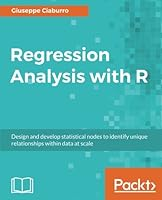# Regression Analysis with R2 Reviews
2018-01-31
422 pages

## Book Description

Regression Analysis with R: Design and develop statistical nodes to identify unique relationships within data at scale

Build effective regression models in R to extract valuable insights from real data

### Key Features

• Implement different regression analysis techniques to solve common problems in data science - from data exploration to dealing with missing values
• From Simple Linear Regression to Logistic Regression - this book covers all regression techniques and their implementation in R
• A complete guide to building effective regression models in R and interpreting results from them to make valuable predictions

### Book Description

Regression analysis is a statistical process which enables prediction of relationships between variables. The predictions are based on the casual effect of one variable upon another. Regression techniques for and analyzing are employed on large set of data in order to reveal hidden relationship among the variables.

This book will give you a rundown explaining what regression analysis is, explaining you the process from scratch. The first few chapters give an understanding of what the different types of learning are - supervised and unsupervised, how these learnings differ from each other. We then move to covering the supervised learning in details covering the various aspects of regression analysis. The outline of chapters are arranged in a way that gives a feel of all the steps covered in a data science process - loading the training dataset, handling missing values, EDA on the dataset, transformations and feature engineering, model building, assessing the model fitting and performance, and finally making predictions on unseen datasets. Each chapter starts with explaining the theoretical concepts and once the reader gets comfortable with the , we move to the practical examples to support the understanding. The practical examples are illustrated using R including the different packages in R such as R Stats, Caret and so on. Each chapter is a mix of theory and practical examples.

By the end of this book you will know all the concepts and pain-points related to regression analysis, and you will be able to implement your learning in your .

### What you will learn

• Get started with the journey of data science using Simple linear regression
• Deal with interaction, collinearity and other problems using multiple linear regression
• Understand diagnostics and what to do if the assumptions fail with proper analysis
• Load your dataset, treat missing values, and plot relationships with exploratory data analysis
• Develop a perfect model keeping overfitting, under-fitting, and cross-validation into consideration
• Deal with classification problems by applying Logistic regression
• Explore other regression techniques - Decision trees, Bagging, and Boosting techniques
• Learn by getting it all in action with the help of a real world case study.

### Who This Book Is For

This book is intended for budding data scientists and data analysts who want to implement regression analysis techniques using R. If you are interested in statistics, data science, and wants to get an easy introduction to the topic, then this book is what you need! Basic understanding of statistics and math will help you to get the most out of the book. Some experience with R will also be helpful

Chapter 1 Getting Started with Regression
Chapter 2 Basic Concepts - Simple Linear Regression
Chapter 3 More Than Just One Predictor - MLR
Chapter 4 Logistic Regression
Chapter 5 Data preparation
Chapter 6 Avoiding Overfitting Problems - Achieving Generalization
Chapter 7 Going Further with Regression Models
Chapter 8 Beyond Linearity - When Curving Is Much Better
Chapter 9 Regression Analysis in Practice

## Book Details

• Title: Regression Analysis with R
• Author:
• Length: 422 pages
• Edition: 1
• Language: English
• Publisher:
• Publication Date: 2018-01-31
• ISBN-10: 178862730X
• ISBN-13: 9781788627306